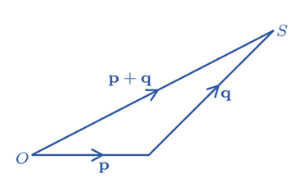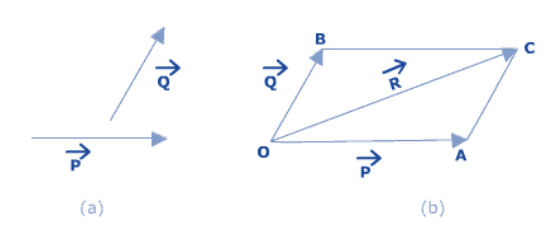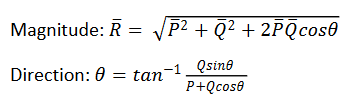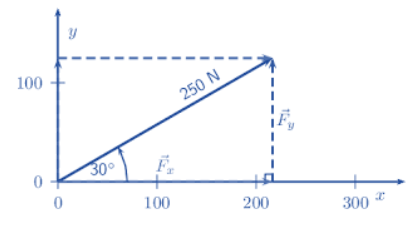# Scalars and Vectors

Scalars and Vectors

Scalar Quantities can be denoted by a number and a unit, i.e. Magnitude. Vector Quantities are denoted by magnitude and direction.

Tensor Quantities

Tensor Quantities are those quantities whose magnitude changes based upon the direction in which you measure it. (e.g. Moment of Inertia)

Types of Vectors

Equal Vectors: Vectors having same direction and magnitude.

Parallel Vectors: Vectors having same direction.

Anti-Parallel Vectors: Vectors having opposite directions.

Position Vector: Vector drawn from the origin to a point.

Zero Vector: Vector of magnitude zero.

Free Vector: Vector which can be moved through space, without changing its direction.

Triangle Law

If two vectors exist such that their directions are taken in order, then their resultant is equal to the third of the triangle formed; & the direction is from head of first to tail of second.Parallelogram Law

The parallelogram law of Vectors states that if two vectors originate or meet at a common point, then their resultant is given by the diagonal of parallelogram formed.Scalar Multiplication

Scalar multiplication refers to the multiplication of a vector with a number.

In Scalar Multiplication, the number is multiplied to all components of the vector. Thus, scalar multiplication does not change the direction of the vector, it only affects the magnitude.

Resolution of Vectors

for co-ordinate system, we know that:

x = rcosθ y = rsinθ

for 3-d coordinate system, we resolve using direction cosines:

r = acosα + acosβ + acosγScalar Product

a.b = abcosθ

Scalar Product gives us a Scalar result.

Vector Product

axb = absinθ

Vector product gives us a vector result.

Unit Vector

A unit vector has unit magnitude and any direction. If we divide a vector by its magnitude, we get an unit vector.

Post By : Ravindra Yadav 02 Nov, 2019 2814 views Physics